# Catch up on the latest AI articles# Frame Interpolation X MAML: Meta-Learning Of Frame Interpolation Models Based On MAML

3 main points
✔️ Apply MAML framework to frame interpolation to respond quickly to new scenes
✔️ Suggest new MAML findings to change data distribution in Innerloop and Outerloop
✔️ Improved PSNR values over baseline in various frame interpolation models with light computational complexity

written by Myungsub Choi, Janghoon Choi, Sungyong Baik, Tae Hyun Kim, Kyoung Mu Lee
(Submitted on 2 Apr 2020)
Subjects: Computer Vision and Pattern Recognition (cs.CV)code：## First of all

Frame interpolation is a technique to make images look smooth. By interpolating images between frames, a choppy image can be made smooth. In practice, a computer creates a continuous intermediate image from the previous and next images softly. When converting low-fps video to high-fps video, frame interpolation is basically the core. Such frame interpolation is one of the CV fields with a long history, but nowadays it is common to compute intermediate images by CNN. Until the advent of SuperSloMo, published by NVIDIA researchers, it was common to input two images to the model and output one intermediate image. Since the advent of SuperSloMo, however, models that output more than one arbitrary intermediate image have been developed.

However, existing highly accurate frame interpolation models are very large and require additional computation time when training new task data. In addition, fine-tuning and other methods to solve this problem have not been studied much.

In "Scene-Adaptive Video Frame Interpolation via Meta-Learning", a method for meta-learning of frame interpolation models is proposed. In this paper, the authors propose a method for meta-learning of frame interpolation models by applying the MAML framework, which has recently attracted much attention in the meta-learning community, to frame interpolation. In this article, we will discuss this paper in detail. (Note that the images in this article are taken from the MAML paper for MAML. All other images are taken from the SAVFI paper)

MAML (Model-Agnostic Meta-Learning ) is a model-independent meta-learning technique. Specifically, MAML is a parameter update technique consisting of an inner loop and an outer loop to make the model adapt to new tasks. After that, the actual parameter update is performed by the Outer loop.

### Inner loop

Suppose a model is $f_\theta$ ($\theta$ is a learning parameter), and $T_{i}$ is a new task for learning. Let $\alpha$ be the learning rate. Then Inner Loop is calculated for each task by the following equation for each task and derives $\theta'_{i}$ by updating parameters for the gradient of each task. This can be done not only by updating once but also by updating an arbitrary number of times.### Outer loop

Then, in the Outer loop, the gradient of each task is summarized and updated in the outer loop for the model that has been updated an arbitrary number of times in the Inner loop. If $\beta$ is the learning rate of the Outer loop, it is defined as below.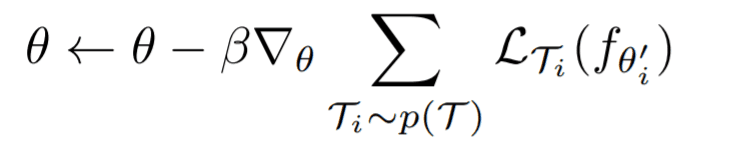For the loss, we take the loss updated for each task in the inner loop, but the update itself uses $\theta$ before the inner loop update, not $\theta'_i$. This is the basic idea of MAML, but please refer to the MAML paper for the mathematical details. The MAML described above suggests that it can be applied to various tasks independent of the model.

## Framework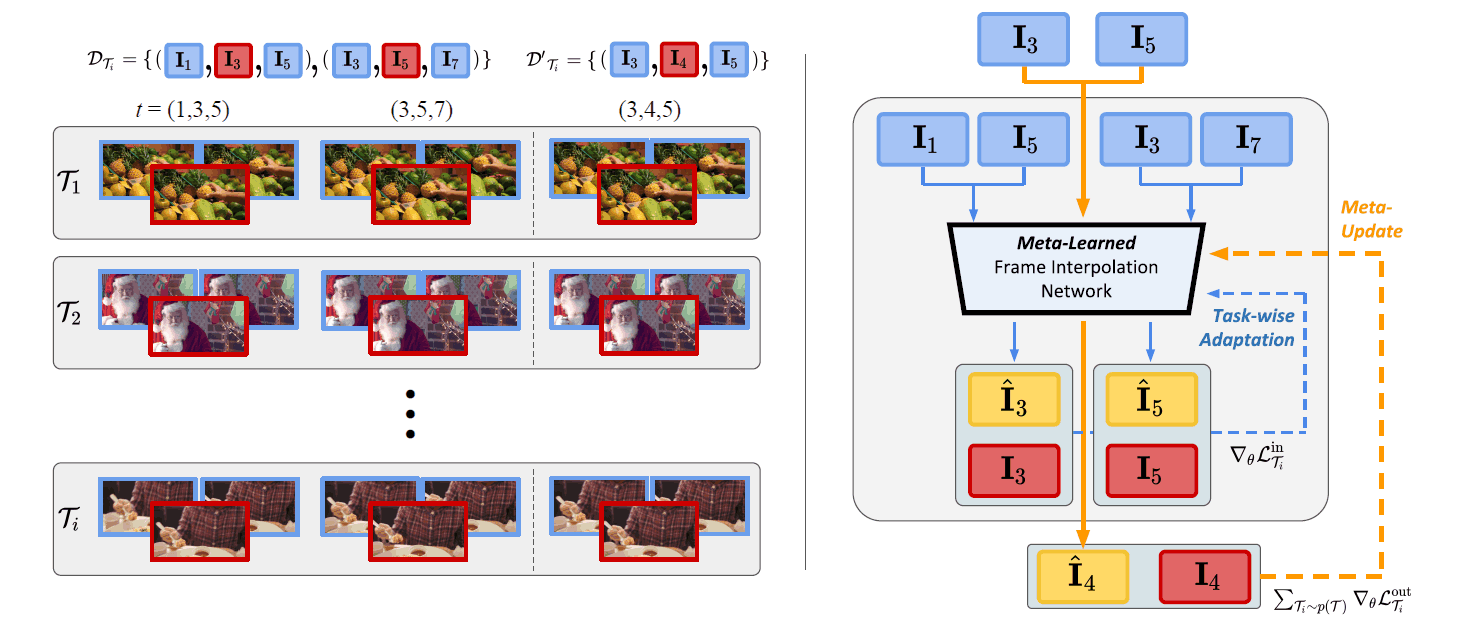In the SAVFI paper, we apply the MAML framework to frame interpolation. The concrete framework is shown in the figure above. The video of the new task is defined as $T_i$. The frame counties to be sampled from each video are defined as $D_{T_{i}}I$ and $D'_{T_{i}}I$, respectively. The former is the data for updating the Inner Loop, and the latter is the data for updating the Outer Loop. In the original paper, the difference from conventional MAML is shown as follows.

Note that, the biggest difference from our algorithm from the original MAML is that the distributions for the taskwise training and test set, $D_{T_{i}}$ and $D_{T_{i}}$, are not the same. Namely, $D_{T_{i}}$ have a broader spectrum of motion and includes $D_{T_{i}}$ , since the time gap between the frame triplets are twice as large.

( "Scene-Adaptive Video Frame Interpolation via Meta-Learning", p6)

In other words, the handling of data with different frame intervals in the Inner Loop and Outer Loop is an unexplored area.

### MAML x Frame Interpolation

In the right figure of the framework, the flow of the update process is described in detail. First, in the inner loop By inputting $D_{T_{i}}I$ into the frame interpolation model $f_{\theta}$, the following interpolated images $\hat{I}_{3}$, $\hat{I}_{5}$ are generated as follows. Then, the inner loop calculates the loss between the interpolated image and the GT for each task.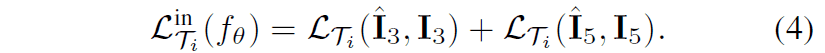After updating the model in the Innerloop described above, the loss in the Outerloop is calculated for each task for the model updated in the Innerloop as in conventional MAML. In addition, the video used for evaluation in the Outerloop is the one with a close frame interval The video used for evaluation in the Outerloop is $D'_{T_{i}}I$ with close frame intervals.The following figure shows the pseudo-code written based on the above two internal and external losses. You can see that the above losses are applied to the MAML framework, and the parameters are updated.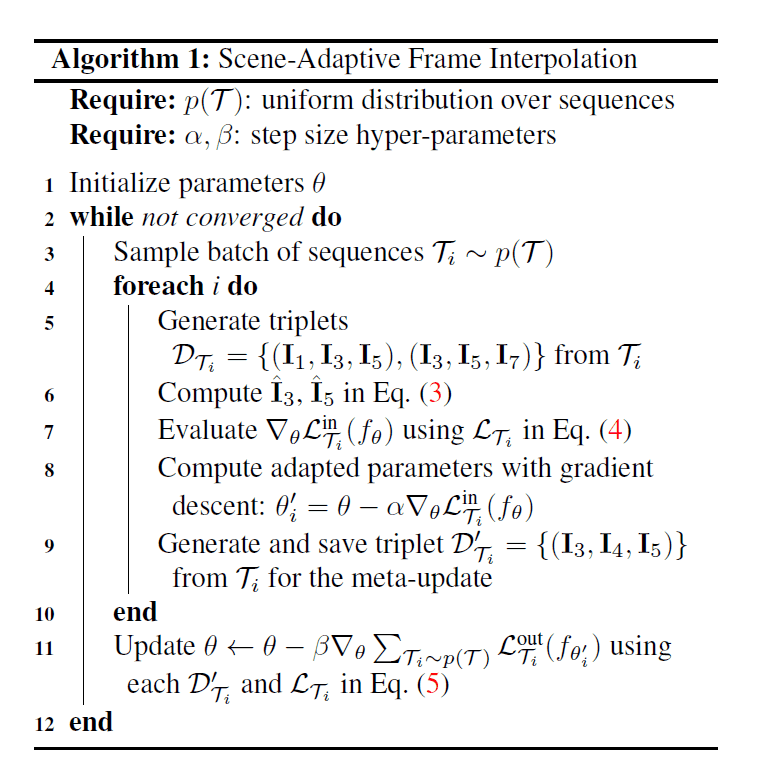## Experimental results

In this paper, the experiments of meta-learning are performed on four models, DVF, SuperSloMo, SepConv, and DAIN, as baselines. As the training data for meta-learning, a train-split of VimeoSeptuplet is used. For the evaluation, the test-split of VimeoSeptuplet and Middlebury-others, HD are used. In addition, the model with the arbitrary number of interpolations like SuperSLoMo is also tested with one interpolation in order to put it into the framework. Specifically, the following three experiments are conducted.

• Quantitative results of existing frame interpolation models by Meta-learning
• Qualitative results of frame interpolation for each data set
• Ablation results for gradient update and learning rate of InnerLoop.

### Quantitative results of existing frame interpolation models by Meta-learning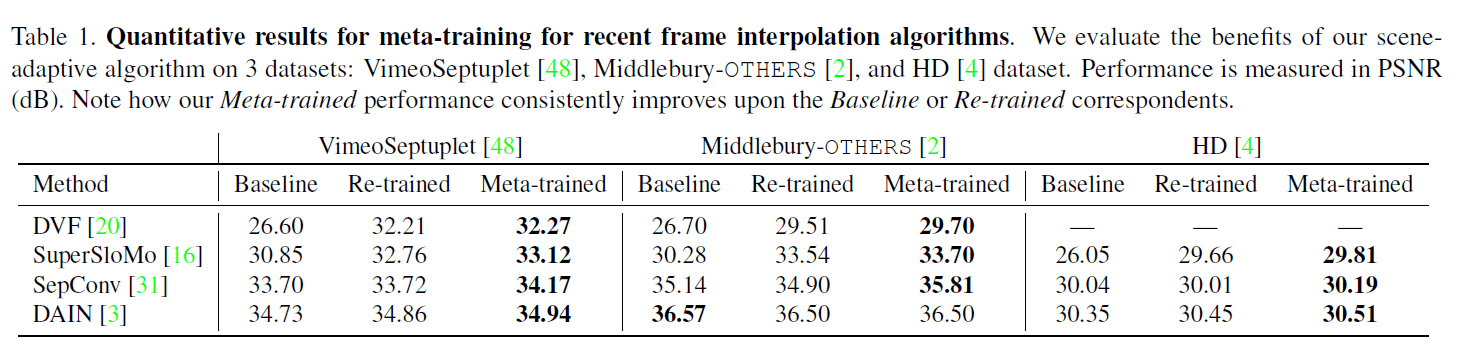The score in the table is the PSNR between the images, and higher values generally mean that the images are closer together. Re-train is simply the result of finetuning using VimeoSeptuplet's train-split. Looking at the bolded area, we can see that the performance of each model is improved against the test-split of VimeoSeptuplet. It can also be confirmed that Meta-learning is effective for most of the models on the other datasets.

### Qualitative results of frame interpolation for each data set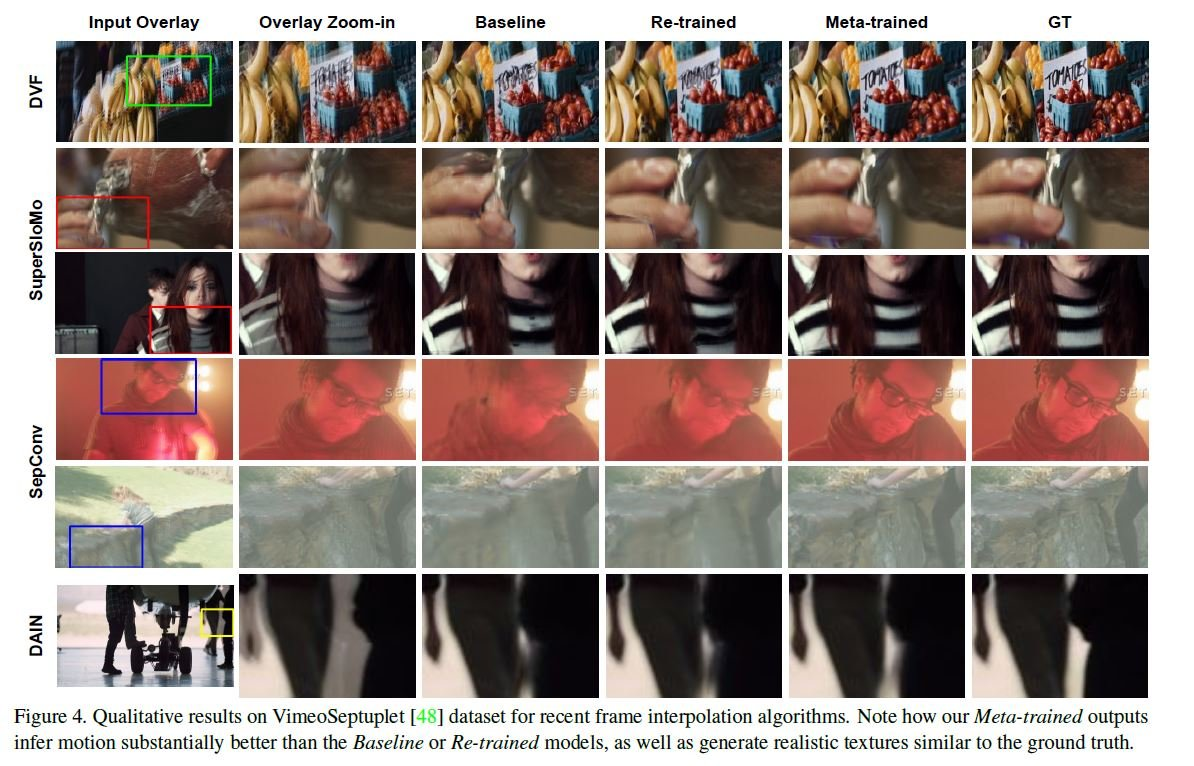The qualitative results are shown in the figure below. The interpolation result of each row of the interpolated image by Meta-learning is clearer and closer to Ground Truth than the other rows. Especially, SepConv is remarkable, and it can be confirmed that the blur is reduced. For more information about SepConv, please refer to Other detailed results on the HD dataset The results also show that It can be confirmed that the sharpness of the interpolated image is improved. The reason for this is that in the paper SepConv's This may be due to the fact that SepConv does not have an optical flow warp transformation mechanism.

### Ablation results for gradient update and learning rate of InnerLoop.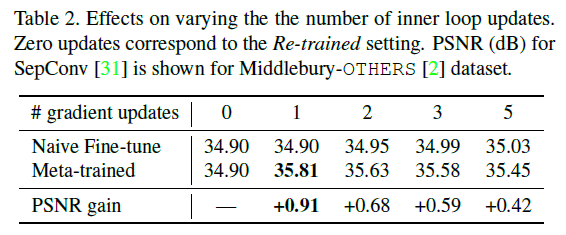This is an ablation of the meta-learning Innerloop with multiple updates, meaning that the single update has the highest PSNR score. This is a different result from the conventional MAML, which is mentioned in the paper as the possibility that multiple updates of the Inner loop overlearn each task and the possibility that the learning becomes more complex.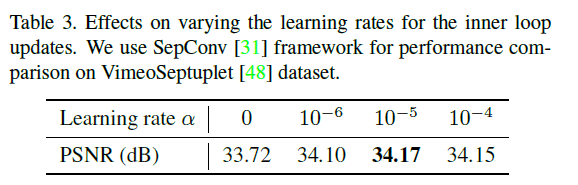This is the result when the learning rate of Innerloop is changed. We can confirm that the best accuracy is obtained by heuristically adjusting the learning rate to the optimal value, which is neither too high nor too low, just like the number of updates above.

## Summary

In this article, we have looked at a paper that applies a frame interpolation task to the MAML framework, which not only demonstrates the versatility of MAML but also explores new possibilities of MAML by using pre-trained models and the nature of the data sampled from the task. The author of the article felt that the use of pre-trained models and the nature of the data sampled from the task led to the exploration of new possibilities for MAML. In conventional interpolation models, there is a tendency to focus only on what kind of flow should be calculated and what kind of network should be derived from it, but the appearance of this paper gives an impression that a new viewpoint is brought to the frame interpolation field.

If you have any suggestions for improvement of the content of the article,